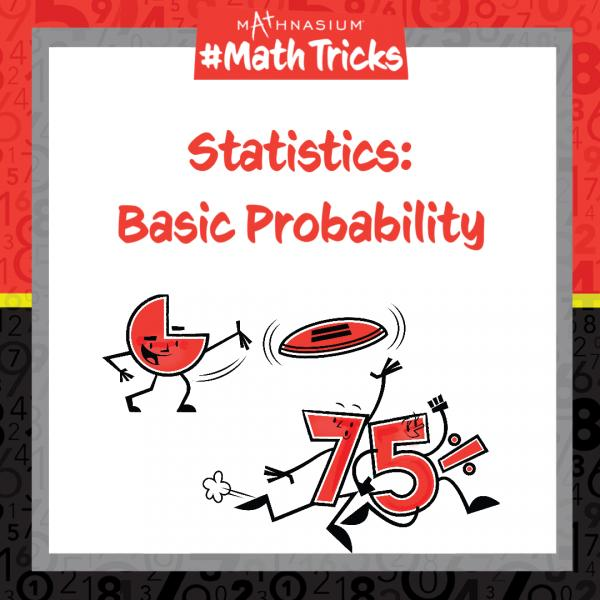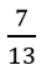# Mathnasium #MathTricks: Probability (Part 1)

By Mathnasium | Added Sep 28, 2022Welcome to Mathnasium’s Math Tricks series. Today we are calculating basic probability.

Probability is the chance or likelihood that something will happen. To calculate the basic probability of something happening, we make a fraction of the number of ways an event can happen (the “favourable” outcomes) out of the total number of “possible” outcomes. Probability can also be represented as a decimal or percent.

The likelihood that something will happen falls between 0 or 0%, (impossible events), and 1 or 100%, (certain events). All other probabilities fall in between 0 and 1.Follow the examples below to find the basic probabilities of the events.

### Step 1: Find the total number of possible outcomes. There is a total of: 3 red marbles + 4 white marbles + 6 blue marbles = 13 marbles.

Step 2: Find the total number of favourable outcomes.
The favorable outcome is pulling a red OR white marble from the bag at random.
The total number of favourable outcomes is: 3 red + 4 white marbles = 7 marbles.

Answer:## Example 2: If a blue marble was pulled from the same bag and not replaced, what is the probability of drawing another blue marble?

Step 1: Find the total number of possible outcomes.
Since a blue marble was pulled from the same bag, there are now: 13 - 1 = 12 marbles.

Step 2: Find the total number of favourable outcomes.
The favourable outcome is drawing another blue marble.
The total number of favourable outcomes is: 6 - 1 = 5 blue marbles.

Answer:Now, with this strategy, you are ready to calculate the basic probability of an event happening. Click here for more practice problems, then check your answers here.

If you missed this, or any of our other Math Tricks videos, check them out on our YouTube channel!